×

Search anything:

# Sorting a 2 Dimensional (2D) vector in C++

#### Software Engineering C++Get this book -> Problems on Array: For Interviews and Competitive Programming

Vectors are data structures similar to array but with features such as dynamic memory, resizable container, insertion and deletion of elements. Vector elements are places in contiguous storage so that they can be accessed and traversed using iterators. A 2D vector is vector of vectors. It is an matrix implemented with the help of vectors.

In this article, we will explore different ways to sort a 2 dimensional (2D) vector in C++ which includes sorting by row, column, a specific row or a specific column.

# Declaration/Syntax:

Vectors are declared in C++ as:
Here 'v' is the name of 1D vector and 'int' is the data type stored.

``````vector<int> v;
``````

2D vectors are defined as:
Here 'v' is the name of vector of vectors(2D vector) and 'int' is the data type

``````vector<vector<int> > v;
``````

We can fill up values in the vectors during initialization or using `push_back()` operation.`push_back()` operation appends the value passed in the function to the end of the vector.

# Sorting types:

### 1. Sorting particular row (Ascending order):

In C++, we can sort particular rows in the 2D vector using `sort()` function, by default the `sort()` functions sorts the vector in ascending order.

Here's a code example(in parts):
Including header files necessary for the vector and sort operations, `bits/stdc++.h` is the header file comprised of several header files and is useful for many operations/functions.

``````#include<bits/stdc++.h> //For 2D vector and sort() function
using namespace std;
``````

Starting of main code:

• Initializing a 2D Vector with random values:
``````    // Initializing 2D vector "vect" with
// values
vector< vector<int> > vect{{6, 6, 1, 4},
{7, 1, 6, 5},
{8, 3, 9, 2}};
``````
• Printing or showing the output of the vector before sorting, here we'll use
`auto` keyword to create a container variable `x` which will take the values of each row in the vector `vect` and then we will create another container variable `y` which takes up each number in `x` and show the output.
``````    // Printing before sorting
// For each element 'x' in 'vect'
for(auto x:vect){
//For each element 'y' in 'x'
for(auto y:x) cout << y << " ";
cout << endl;
}
//OUTPUT:
// 6 6 1 4
// 7 1 6 5
// 8 3 9 2
``````
• `sort()` is an inbuilt function in the C++ STL library, this function takes the starting address of the vector to sort and the ending address of the vector, all element between starting and ending addresses gets sorted according to the preference. Here, we're sorting 2nd row of the vector,
``````    // Use of "sort()" for sorting second row
// Indexing starts from '0'
sort(vect.begin(), vect.end());
``````
• Showing the output after sorting
``````    // Displaying the 2D vector after sorting
cout << "The 2D vector after sorting 2st row is:\n";
for(auto x:vect){
//For each element 'y' in 'x'
for(auto y:x) cout << y << " ";
cout << endl;
}
//OUTPUT:
// 6 6 1 4
// 1 5 6 7
// 8 3 9 2
``````

Here's the pictorial representation of the vector before and after sorting,
Vector before sorting: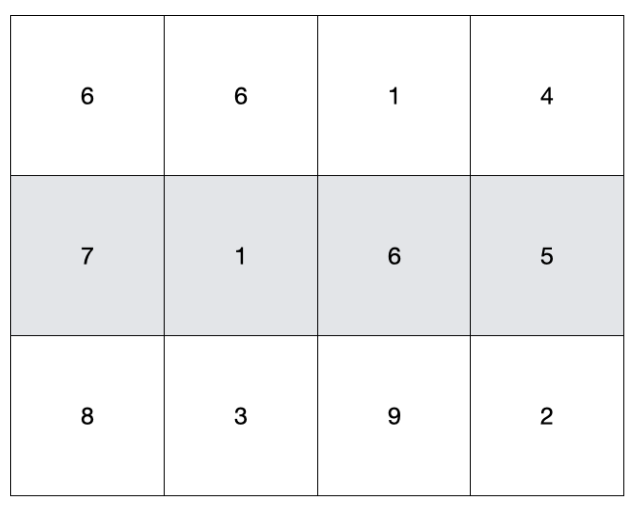Vector after sorting second row: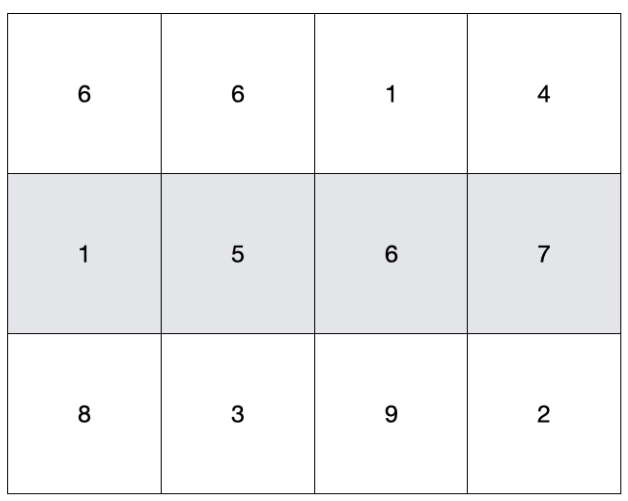### 2. Sorting particular column (Ascending order):

Similary, we can sort the columns using `sort()` function, but when we sort a column, the rows rearrange too.

Here's an example code:

• Including the base library for vector and sort functionality,
``````#include<bits/stdc++.h> //For 2D vector and sort() function
using namespace std;
``````
• For sorting the columns we have to create a function which compares the columns and rearrange rows as to make the order ascending or descending, we are creating a function named `sortcol()` which takes by two vector addresses and returns `true` or `false` based upon the preference.
``````//For comparing 2'nd column order
bool sortcol(const vector<int>& v1,const vector<int>& v2) {
return v1 < v2;
}
``````
• Starting of the main code:
``````int main()
{
...
}
``````
• Again we'll initialize 2D vector with random unsorted values,
``````    // Initializing 2D vector "vect" with
// values
vector< vector<int> > vect{{6, 6, 1, 4},
{7, 2, 6, 5},
{8, 3, 9, 2}};
``````
• Showing each element in the vector before sorting for comparision,
``````    // Printing before sorting
// For each element 'x' in 'vect'
for(auto x:vect){
//For each element 'y' in 'x'
for(auto y:x) cout << y << " ";
cout << endl;
}
//OUTPUT:
// 6 6 1 4
// 7 2 6 5
// 8 3 9 2
``````
• Now we'll use `sort()` function with 3 parameters: starting address, ending address and the preference respectively, here `sortcol()` compares 2 rows and return true or false according to the condition set.
``````    // Use of "sort()" for sorting second row
// Indexing starts from '0'
sort(vect.begin(), vect.end(),sortcol());
``````
• Displaying the contents of vector after sorting columns,
``````    // Displaying the 2D vector after sorting
cout << "The 2D vector after sorting 2nd row is:\n";
for(auto x:vect){
//For each element 'y' in 'x'
for(auto y:x) cout << y << " ";
cout << endl;
}
//OUTPUT:
// 7 2 6 5
// 8 3 9 2
// 6 6 1 4
``````

Here's the visual representation of the vector before and after sorting,
Vector before sorting:Vector after sorting: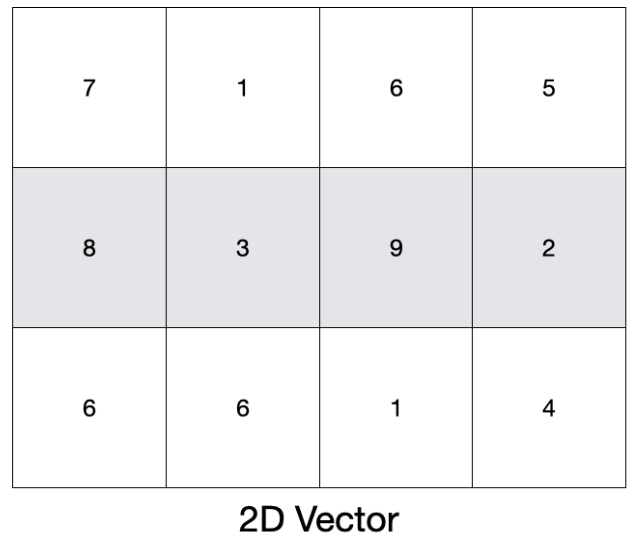### 3. Sorting particular row (Descending order):

We can sort a particular column in descending order by passing the reversed vector pointer(`rbegin()` and `rend()`) in the `sort()` function.

Here's a code example:

• Including base library for vector and sorting functionality,
``````#include<bits/stdc++.h> //For 2D vector and sort() function
using namespace std;
``````
• Starting of `main()` function, all the code goes inside this function
``````int main()
{
...
}
``````
• Initializing the 2D vector with random values
``````    // Initializing 2D vector "vect" with
// values
vector< vector<int> > vect{{6, 6, 1, 4},
{7, 1, 6, 5},
{8, 3, 9, 2}};
``````
• Displaying the vector before sorting for comparision,
``````    // Printing before sorting
// For each element 'x' in 'vect'
for(auto x:vect){
//For each element 'y' in 'x'
for(auto y:x) cout << y << " ";
cout << endl;
}
//OUTPUT:
// 6 6 1 4
// 7 1 6 5
// 8 3 9 2
``````
• We'll again use the `sort()` function, but this time we'll pass the reverse address for starting and ending positions, by that the vector will be sorted in ascending order but reversed as to make it descending,
``````    // Use of "sort()" for sorting second row
// Indexing starts from '0'
sort(vect.rbegin(), vect.rend());
``````
• Displaying the 2D vector after sorting to compare with previous results.
``````    // Displaying the 2D vector after sorting
cout << "The 2D vector after sorting 2nd row is:\n";
for(auto x:vect){
//For each element 'y' in 'x'
for(auto y:x) cout << y << " ";
cout << endl;
}
//OUTPUT:
// 6 6 1 4
// 7 6 5 1
// 8 3 9 2
``````

Here's the visual representation of the 2D vector before and after sorting row,
Vector before sorting: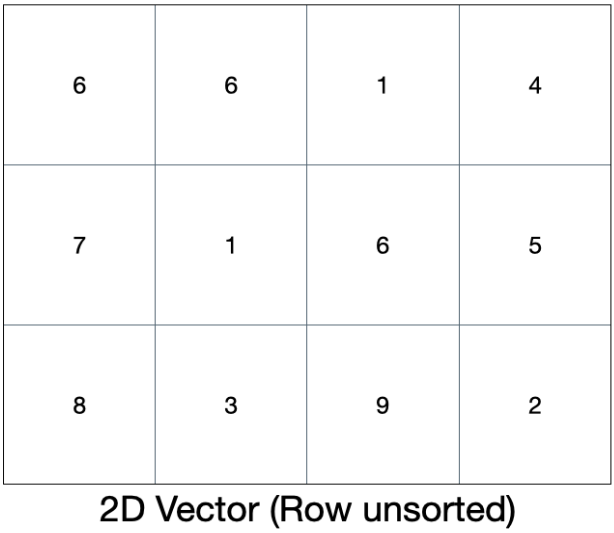Vector after sorting: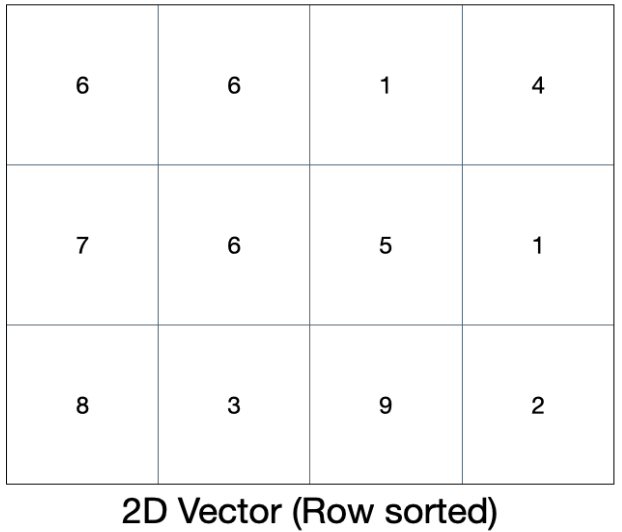### 4. Sorting particular column (Descending order):

Similarly, we can sort the column by using `sort()` function but by modifying the `sortcol` function to compare greater elements.

Here's a code example:

• Including the base library for vector and sort functionality,
``````#include<bits/stdc++.h> //For 2D vector and sort() function
using namespace std;
``````
• As the columns can't be sorted by the `sort()` funtion alone, we'll create a `sortcol()` function which takes two vector rows and compare them in descending order.
``````//For comparing 2nd column order
bool sortcol(const vector<int>& v1,const vector<int>& v2) {
return v1 > v2;
}
``````
• Starting of the `main()` function, all the executable code goes here
``````int main()
{
...
}
``````
• Initializing 2D vector with random unsorted values,
``````    // Initializing 2D vector "vect" with
// values
vector< vector<int> > vect{{6, 6, 1, 4},
{7, 2, 6, 5},
{8, 3, 9, 2}};
``````
• Displaying the contents of the vector before sort for comparision,
``````    // Printing before sorting
// For each element 'x' in 'vect'
for(auto x:vect){
//For each element 'y' in 'x'
for(auto y:x) cout << y << " ";
cout << endl;
}
//OUTPUT:
// 6 6 1 4
// 7 1 6 5
// 8 3 9 2
``````
• Here we'll use the `sort()` function with 3 arguments: starting address, ending address and the `sortcol()` function for comparision(descending order).
``````    // Use of "sort()" for sorting second column
// Indexing starts from '0'
sort(vect.begin(), vect.end(),sortcol());
``````
• Displaying the 2D vector after sorting 2nd column,
``````    // Displaying the 2D vector after sorting
cout << "The 2D vector after sorting 2nd column is:\n";
for(auto x:vect){
//For each element 'y' in 'x'
for(auto y:x) cout << y << " ";
cout << endl;
}
//OUTPUT:
// 6 6 1 4
// 8 3 9 2
// 7 1 6 5
``````

Here's the visual representation of vector before and after sorting,

Vector before sorting: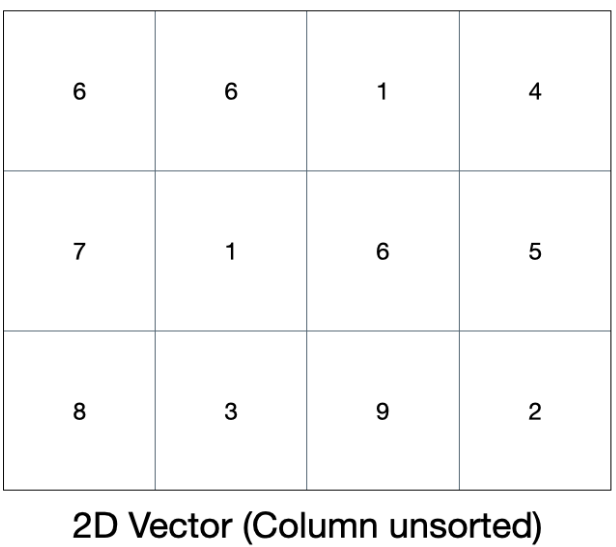Vector after sorting: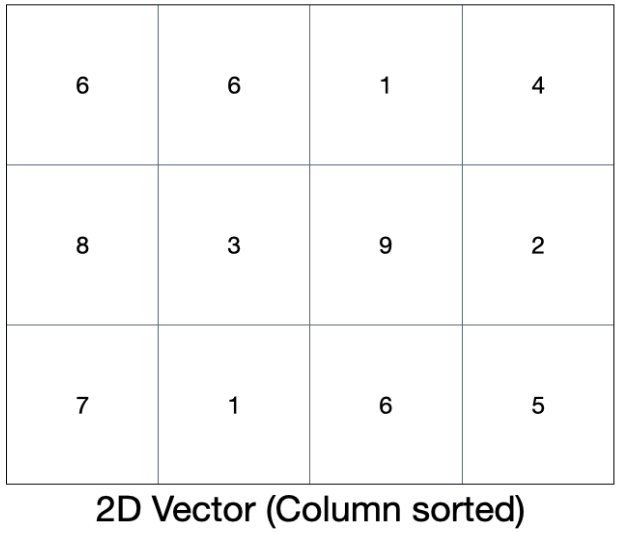With this article at OpenGenus, you must have the complete idea of sorting a 2D vector in C++. Thank you for reading this post!#### Sahil Silare

B.Tech Student in Computer Science at National Institute of Technology (NIT), Raipur (2019 - 2023) | Intern at OpenGenus

Sorting a 2 Dimensional (2D) vector in C++